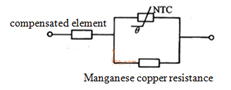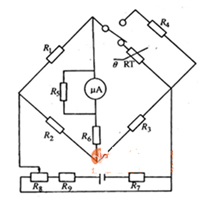# How to use the NTC

Time : Mar 02 2015Source :analogtechnologies Author : Analog Click :

The NTC element is a negative temperature coefficient thermistor, which is widely used in radio production. This paper mainly introduces the applications of the following three aspects.
1. Temperature compensation in instrument circuit
In the instrument circuit, there are a lot of elements with metal wire, which is like wire wound resistance. As metal wire generally has a positive temperature coefficient, using NTC for temperature compensation, errorsproduced bytemperature changes can be offset. Figure 1 is a temperature compensation circuit. NTC is connected in parallel with manganese copper resistance, and then it is in series with the compensating elements, so the function of temperature compensation can be achieved.Fig. 1 Application of NTC in instrument temperature compensation

2. Steady working point of NTC in the transistor circuit
Figure 2 is a circuit of stable transistor working point of three kinds of NTC.
Figure 2 (a) shows that a simple transistor current amplifier took a big RT in the base loop. When temperature changes in the environment, line output current will change. And after increasing NTC, the collector current can be automatically adjusted and the output gain can be stable.
In the fig 2(b), the NTC is connected in parallel withemitter resistance. When theresistance of transistor emitter junction increases with the temperature increasing, the RT will play the role of compensation.
Figure 2 (C) shows low frequency power amplifier stage of a transistor radio. The under bias resistor of this levelis connected in parallel with on RT.When the temperature rises cause electrode current increasing, the under bias resistance will decrease and the base current will also reduce, so the collector current can be fall, which plays the role of stabilizing operating point.Fig. 2 Steady working point of NTC in the transistor circuit

3 The temperature measuring device with NTC
What is shown by Figure 3 is a thermistor thermometer. In the figure, the RT is thermistor.As the resistance change of the thermistor is with temperature changes, micro ammeter will change accordingly, which is connected between the bridge diagonal. Thermistor thermometer accuracy can reach 0.1℃, and the temperature sensitivity is under 10s.Fig. 3 Thermistor thermometer